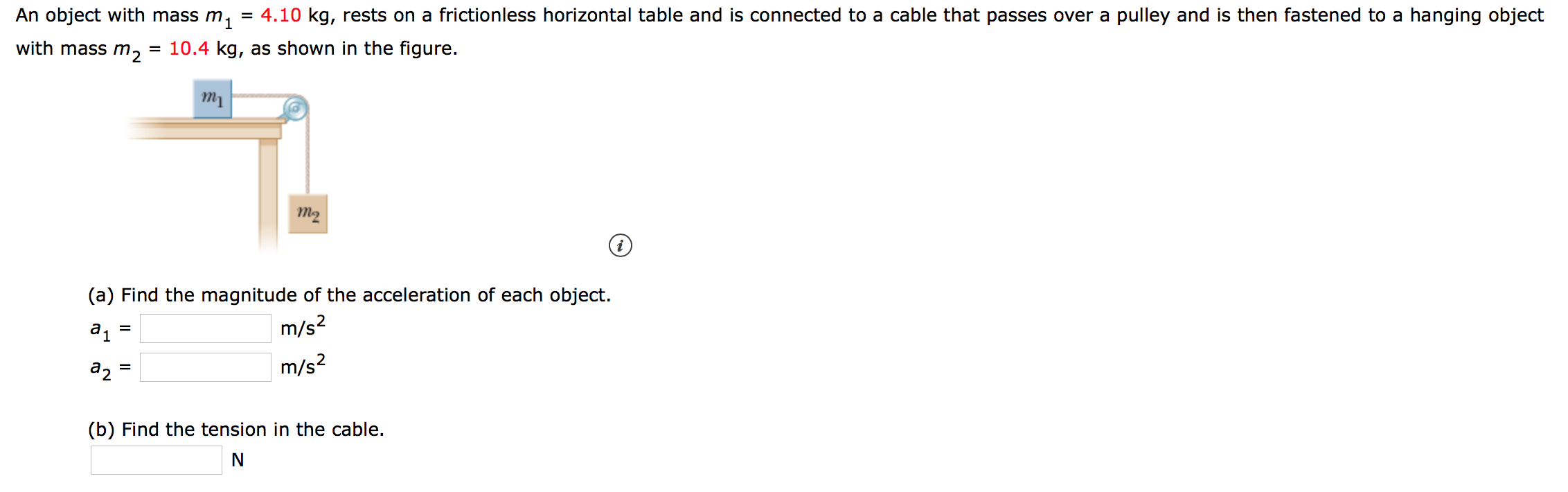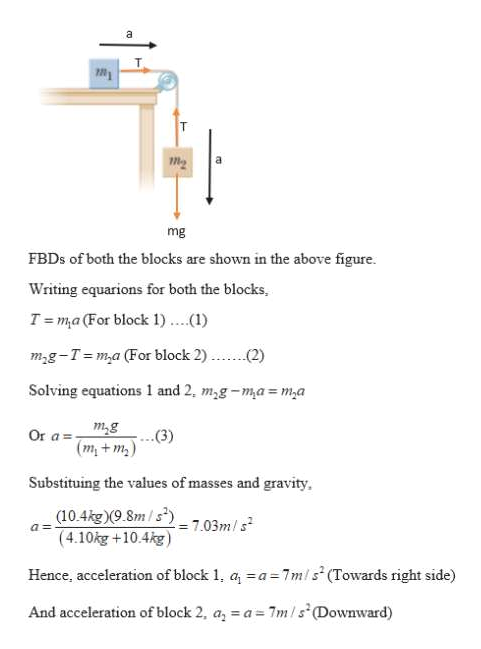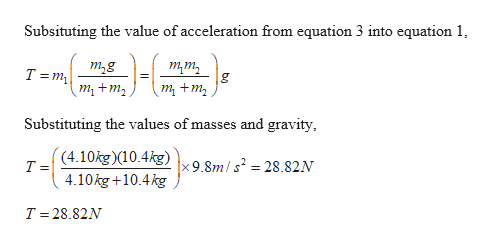# = 4.10 kg, rests on a frictionless horizontal table and is connected to a cable that passes over a pulley and is then fastened to a hanging objectAn object with mass m.1with mass m = 10.4 kg, as shown in the figureтyт2(a) Find the magnitude of the acceleration of each objectm/s2а1m/s2а2(b) Find the tension in the cableN

Question
4 viewshelp_outlineImage Transcriptionclose= 4.10 kg, rests on a frictionless horizontal table and is connected to a cable that passes over a pulley and is then fastened to a hanging object An object with mass m. 1 with mass m = 10.4 kg, as shown in the figure тy т2 (a) Find the magnitude of the acceleration of each object m/s2 а1 m/s2 а2 (b) Find the tension in the cable N fullscreen
check_circle

Step 1

Given:

Mass of the first block, m1 = 4.10 kg

Mass of the second block, m2 = 10.4 kg

Step 2

(a) Calculating the accelerations of the blocks:

As both blocks are tied with the same strong inextensible string, so acceleration of both the blocks would be the same. Let’s assume acceleration of the blocks is ‘a’ as shown.help_outlineImage Transcriptioncloseа mg FBDs of both the blocks are shown in the above figure Writing equarions for both the blocks, T ma (For block 1)..) (2) mg-T=m,a (For block 2) Solving equations 1 and 2, m,g-ma m,a mg (my+ m Or a= (3) Substituing the values of masses and gravity (10.4kg)(9.8m/s) (4.10kg +10.4kg) = 7.03m/s a= Hence, acceleration of block 1, a a 7mls (Towards right side) And acceleration of block 2, a, a=7m/s (Downward) fullscreen
Step 3

(b) Calculating the tensi...help_outlineImage TranscriptioncloseSubsituting the value of acceleration from equation 3 into equation 1 -6 т,g uш т +m, T m т +m, Substituting the values of masses and gravity (4.10kg)(10.4kg) T = x 9.8m/s2 = 28.82N 4.10kg+10.4kg T 28.82N fullscreen

### Want to see the full answer?

See Solution

#### Want to see this answer and more?

Solutions are written by subject experts who are available 24/7. Questions are typically answered within 1 hour.*

See Solution
*Response times may vary by subject and question.
Tagged in

### Newtons Laws of Motion